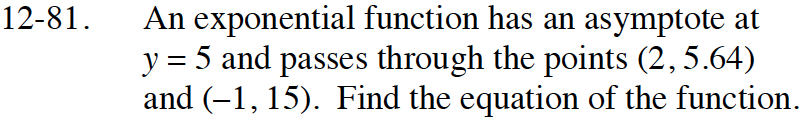Home > CCA2 > Chapter 12 > Lesson 12.1.4 > Problem12-81

12-81.

An exponential function has an asymptote at y = 5 and passes through the points (2, 5.64) and (−1, 15). Find the equation of the function. Homework Help ✎Use y = abx + k.

y = 4(0.4)x + 5